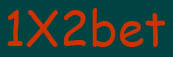Let's consider some simple examples of rates.

In a daily line the list of events on which rates are accepted is resulted. You can choose any event and count on any outcome according to rules. The rate can be "single", "express train" or "system".

Let's admit, we were interested by matches of the championship of Spain on football:

 Ή Date Command 1 Command  2 1 Υ 2 1Υ 12 Υ2 Τ1 Κ1 Τ2 Κ2 Total < > 1 12.12.2002 Real Madridε Seville 1,22 4,90 13,65 1,02 1,10 3,45 -2 2,00 +1,5 2,15 2,5 2,00 1,70 2 12.12.2002 Deportyvo Barselona 1,66 3,36 4,87 1,11 1,25 2,04 -1 2,06 +1 1,64 2,5 1,95 1,75 3 12.12.2002 Selta Recreativoε 1,50 3,55 6,25 1,07 1,20 2,27 -1 1,92 +1 1,80 2,5 1,90 1,80

Rates on outcomes:

1 - a victory of the first command;
Υ - a drawn game;
2 - a victory of the second command;
1Υ - a victory of the first command or a drawn game;
12 - a victory of the first command or a victory of the second command;
Υ2 - A victory of the second command or a drawn game;

Rates with odds:

Τ1 - Odds under rates at the first command;
Κ1 - Factor on the rate at the first command with odds;
Τ2 - Odds under rates at the second command;
Κ2 - Factor on the rate at the second command with odds;
At rates with odds to quantity of goals of the command chosen by you the odds increase. If after addition the chosen command has won, the rate has played, if the drawn game - return (payment with factor 1) if has lost - the rate has not played.
Example. You put for Real Madrid with odds "-2". If the Real wins in 3 balls or more (3:0, 4:0, 4:1 etc.), the rate will play. If the Real wins a victory in 2 balls (2:0, 3:1 etc.) the rate is subject to return. If the Real wins in 1 ball, will draw or οξπθγπΰες, the rate will not play.

Rates on
total:

Example. At rates on total you should guess quantity of the hammered goals (more or less σκΰηΰνξγξ totalΰ). I.e. that the rate on total more than 2,5 has played, in a match should be hammered 3 or more goals (2:1, 2:2, 3:0 etc.). That the rate on total less than 2,5 has played, in a match should be hammered 1 or 2 goals, or the account 0:0 is fixed.
Normal level:
Example. We will assume, you have counted \$10. On a Selta victory. Prize factor under rates on a Selta victory - 1,50. In case of a victory of a Selta payment will make 10 υ 1,50 = \$15.
Net gain: 15 (payment) - 10 (rate) = \$5.

The express train:

Example. We will assume, you have counted \$10. On the express train consisting of three outcomes: Real with odds "-2", Deportivo - Barcelona - a drawn game, a Selta victory. Factors of prizes on the outcomes entering into the express train, are multiplied: 2,00 υ 3,36 υ 1,50 = 10,08. If you have correctly predicted the Real victory in 3 balls or more and both other outcomes, payment will make: \$10. υ 10,08 =
\$100,8., including a net gain (minus the rate sum): \$90,8. If the Real has won Madrid in 2 balls the factor on payment on this outcome becomes equal 1. Thus the factor on payment on the express train will be equal: 1 υ 3,36 υ 1,50 = 5,04. Payment will make: \$10. υ 5,04 = \$50,4., from them a net gain - \$40,4.

System:

Example. We will assume, you have counted
\$15. On system 2 from 3, consisting of three outcomes: Real with odds "-2", Deportivo - Barcelona - a drawn game, a Selta victory. I.e. actually it is the rate on 3 express trains:
The real with odds "-2", - Barcelona - a drawn game, factor is equal to Deportivo 2,00 υ 3,36 = 6,72;
The real with odds "-2", a Selta victory, factor is equal 2,00 υ 1,50 = 3,00;
Deportivo - Barcelona - a drawn game, a Selta victory, factor it is equal 3,36 υ 1,50 = 5,04;
The sum of rates on each express train is equal to the sum to all rate on system, divide on quantity of variants (express trains). The rate sum on each express train is equal In the given example \$5. The payment sum on system is equal to the sum of payments on all express trains. I.e. at the correct forecast for all 3 events it is had:
5 υ 6,72 + 5 υ 3,00 + 5 υ 5,04 = \$73,8. The Net gain: \$58,8.
If forecasts only on 2 events coincide: for example, Deportivo - Barcelona - a drawn game and a Selta victory one express train will play only:
5 υ 5,04 = 25,2. The Net gain: \$10,2. Thus we have profit even having guessed 2 events from 3.